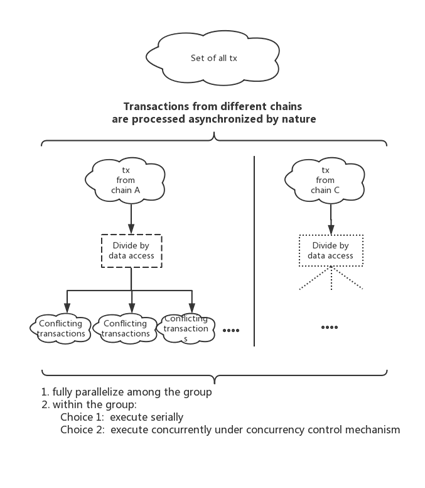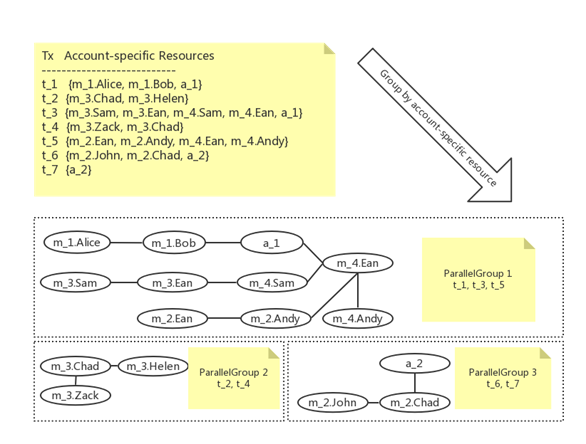# Aelf Tech in Detail — Concurrent Scheduling

By Rong Peng

1. Basic idea

The goal is to design a strategy to execute smart contracts concurrently. It must have good performance when applying the strategy to a cluster environment.

There are two ways to achieve this:

1. Resource Isolation

2. Database Concurrency Control Techniques .

Resource isolation is about detecting and separating the transactions that access different resources. The resource detection actions are performed statically and occur before the contract execution.

Database concurrency control techniques resolve concurrency problem at runtime. It uses a scheduler to receive and deal with the request sent by different contracts. When a new request arrives, the scheduler will make a decision on whether to approve or delay the request based on whether this request conflicts with the current approved requests of the running transactions. Sometimes the scheduler will even abort and restart a transaction if this transaction’s actions violate the transactional requirement.

We use the resource isolation strategy because it fits the requirement for running the transactions on a cluster node. Database concurrency control needs a central scheduler (this “central” has no relation with the “decentralize” aspect of the blockchain) to determine whether a data request conflict with other requests, this means the network latency that occurs when running the transactions on a different host of a cluster is unacceptable.

The database concurrency control is not mutually exclusive, and we can apply specialized database concurrency control techniques within the resource isolated group to further exploit concurrency potential.

The steps of the resource isolation strategy we designed are shown as follows:

# Step 1: Parallel among Transaction Groups

Split the transactions into groups according to the set of the resources that transaction might access (figure 1) so that there will be no conflict among the tx from different groups. Hence, we can fully parallelize among the groups.Figure 1: divide the tx into groups that can run in parallel

# Step 2: Concurrency within the group

[this section is not part of actual design, but is just to provide a better understanding of the idea]

Currently we execute the conflicting group in serial.

Transactions in a blockchain must be executed by transaction and deterministically, which means we must use specialized concurrency control techniques if we want to correctly execute the conflicting transactions concurrently. The rationale behind the combination of these two ideas is the fact that the conflicting groups will be better executed on one computer to avoid network cost during execution. Therefore, we are able to apply concurrency control techniques with only local inter-thread communication, which is much less costly compared with network latency of inter-host communication.

2. Design

# 2.1 Classify data into 3 three classes

We classify contract state data into 3 classes as follows to distinguish different data access scenarios.

1. Read-only account-sharing data — Data that is not going to change in a block’s execution.

Such as “block height”, “prev-block hash” and other user defined read-only constant.

2. Read-write account-sharing data — The data where a variable could be accessed(r/w) by multiple accounts.

Example: Consider a ballot contract, for each proposal in the proposals list to be voted, they could be accessed by multiple accounts (e.g. Alice and Bob both vote for proposal)

3. Account-specific data — It is typically a map where:

(1) An address is the key and the address-related data is the value.

(2) An address can only access its own data.

Example: Consider a token contract, the balance map is account-specific data. A transaction which calls a function where Alice transfers to Bob can never access John’s data in this balance map.

The incentive to classify data is that most of the smart contract’s logic is account-related. In other word, many smart contract functions only access “Account-specific data”. In this case, the transaction about “Alice” and “Bob” and transaction about “Helen and Chad” can run in parallel even if they are operating on the same “Account-specific data”. (Because they access different data items and don’t have data conflicts).

We refer to “a_i” as a read-write account-sharing data and “m_i” as a account-specific data in following examples.

# 2.2 Function’s Metadata

In order to get the set of resources that might be occupied by the transaction, we need to know what resource will be used by each function of every contract. We use a function’s metadata to fulfill that need. The function’s metadata represents the set of resources, i.e. the state data that might be occupied by a contract function.

The metadata contains “calling set” and “resource set”.

Function “calling_set” — every function need to specify “what other function this function will call”

Function “resource_set” — every function need to specify “what data this function will access (for now we don’t separate read and write)”. We use [contract address + resource name] as the full name of the resource, which can be seen as the unique ID of a data item (a variable, a collection). E.g. the full name of “balanceOf” map of “SomeToken” contract with address 0x123 can be “0x123.balanceOf”

NOTE: Function Metadata should be statically analyzed when the contract is created on the blockchain and persist to the database.

For details of the function’s metadata, see doc About metadata （this doc is a work in progress）

# 2.3 How to divide the tx into groups

We can divide the transactions from the same chain in three steps:

1. Extract the resource set of the transaction according to different resource types marked in the metadata.

2. Form a resource dependency graph (undirected graph). In this graph, the node is the resource and there will be an edge between two resources when a transaction accesses these two resources.

3. Divide transactions into different groups according to the resource dependency graph, where transactions in the same connected component are in the same group.

The strategy can be described as follows:

Metadata analysis, determining the resource set of a contract function

[Statical, determined when deploying contract]

|

| — — — -Group transactions by using metadata

[Determined before executing transactions]

|

| — — — -Concurrency control inside group (TBD)

# 2.3.1 Grouping transactions according to resource set

[Basic idea]

Suppose we have transactions “t_1 [Alice, Bob] {m_1}” and “t_2 [Chad, Helen] {m_1}” where m_1 is account-specific data. Then t_1 will access m_1.Alice and m_1.Bob, and t_2 will access m_1.Chad and m_1.Helen. Hence they can run in parallel because they have no conflicts.

For transactions that access the same r/w account sharing data a_1, they will be considered to access the same resource a_1 since multiple accounts can access the same data item in a_1.

[Grouping algorithm]

Step 1: For every transaction, we mark the resource by using the rules below (suppose transaction t_i has related accounts, Alice and Bob):

(a) If t_i might access account-specific resource m_j, then add resource “m_j.Alice” and “m_j.Bob” into the resource set of t_i.

(b) If t_i might access r/w account sharing resource a_k, then add resource “a_k” into the resource list of t_i.

© If t_i might access read-only account sharing resource, ignore and continue.

An example of step 1 is shown in figure 2.

Step 2: Group transactions according to account-specific resources

As we mentioned above, if we have an undirected graph where vertex is resources and there always exists a path between every two resources that will be accessed by a transaction. Then the transactions belonging to the same connected component should be in the same group.

Hence, we use a union-find set to solve this problem by using the following steps:

1. Use the union-find set to build up the supersets of resources of conflicting transactions when scanning the resource set of each transaction.

2. For each transaction, if the resource accessed by this transaction is in the i-th superset, then this transaction should be in the i-th group

The example is shown in figure 3. The 7 transactions are divided into 3 groups where they don’t share account-specific resource (account-specific data & r/w account-sharing data) across the groups.Figure 3: Grouping transactions according to account specific resource

# 2.4 Challenges

1. How to analysis the resource set when a contract calls another contract under the current implementation of contract calling.

2. How to let contract developers mark the metadata correctly. (Coding concurrent programs can easily go wrong and is hard to debug. We are considering implementing a static code analysis tool in the future to narrow the space of human error).

3. How to optimize grouping (granularity, etc.) to keep the grouping time negligible compared with the contract execution when facing large amount of transactions in a block.

# Reference

 Dickerson, Thomas, et al. “Adding concurrency to smart contracts.” Proceedings of the ACM Symposium on Principles of Distributed Computing. ACM, 2017.

 Zhang, An, and Kunlong Zhang. “Enabling Concurrency on Smart Contracts Using Multiversion Ordering.” Asia-Pacific Web (APWeb) and Web-Age Information Management (WAIM) Joint International Conference on Web and Big Data. Springer, Cham, 2018.

--

--